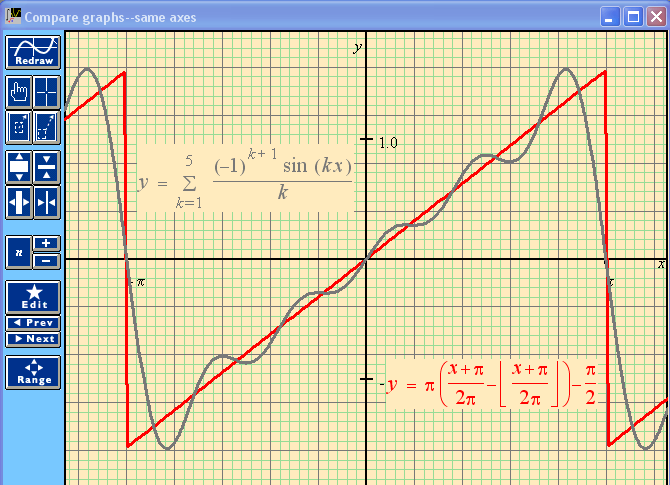# Help With Math: Purveyors of MathXpert

-->This type of graph allows the student to compare functions on the same axes. This example shows the first n terms of a trigonometric series (known as a Fourier series) converging to a "sawtooth function". Clicking the button labeled n will increase the number of terms shown in the graph. (In MathXpert, that is--clicking here won't do anything, this is just a picture of a MathXpert screen.) This particular example illustrates an interesting piece of history. In 1769, James Bernoulli asserted that every function could be written as the sum of an infinite trigonometric series. The famous mathematician Leonhard Euler said that this could not be correct. His argument was this: there are discontinuous functions (like the sawtooth function shown here), but the trigonmetric functions are continuous, and sums of continuous functions are continuous, so how could you get a discontinuous function by adding up continuous functions? The graph above shows how! In MathXpert, you can use the n button on the Graph Toolbar to increase n and watch the series converge to the sawtooth function. You can even see the wiggles at the corners known as the Gibbs phenomenon. If Euler had had MathXpert, he wouldn't have made this mistake, and the knowledge of such series would not have had to wait for Fourier's 1807 publication.